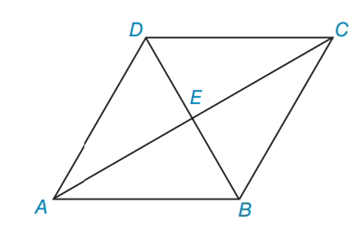Chapter 4.3, Problem 18EElementary Geometry For College St...

7th Edition
Alexander + 2 others
ISBN: 9781337614085

Solutions

Chapter
SectionElementary Geometry For College St...

7th Edition
Alexander + 2 others
ISBN: 9781337614085
Textbook Problem

In Exercises 15 to 18, consider rhombus ABCD with diagonals A C ¯  and  D B ¯ . When the answer is not a whole number, leave a square root answer.Exercises 15 – 18If AC = 14 and DB = 10, find BC.

To determine

To find:

BC of the given rhombus.

Explanation

The basic properties of rhombus:

1) All the properties of a parallelogram apply (the ones that matter here are parallel sides, opposite angles are congruent, and consecutive angles are supplementary).

2) All sides are congruent by definition.

3) The diagonals bisect the angles.

4) The diagonals are perpendicular bisectors of each other.

Calculation:

Given: AC = 14 and DB = 10

Consider the rhombus.

To find BC:

From the property, in rhombus, two diagonals are perpendicular bisectors of each other.

In rhombus ABCD,

AE=EC=AC2=142=7

DE=EB=DB2

Still sussing out bartleby?

Check out a sample textbook solution.

See a sample solution

The Solution to Your Study Problems

Bartleby provides explanations to thousands of textbook problems written by our experts, many with advanced degrees!

Get Started

Solve the equations in Exercises 126. 14x2=0

Finite Mathematics and Applied Calculus (MindTap Course List)

In Exercises 3-6, simplify the expression. 12(3)x232x1/2

Calculus: An Applied Approach (MindTap Course List)

Prove the identity. 55. sin1cos=csc+cot

Single Variable Calculus: Early Transcendentals

Find for x = 3t2 + 1, y = t6 + 6t5. t4 + 5t3 4t3 + 15t2

Study Guide for Stewart's Multivariable Calculus, 8th